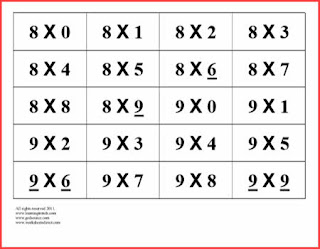## Friday, December 07, 2018

### Do I Really Need to Study Math?

For a student who simply wants to "pass" the GED, it is tempting to spend little time on the math portion. For some students, those who have a fairly good foundation in math concepts, that is ok. But for those students who don't have a good foundation, this can be disastrous. Those students typically want to "bounce" around from topic to topic.
When tutoring my GED math students, I use an analogy of a mason building a brick house. Each brick represents a math concept. You must first lay a solid foundation of basic whole number understanding. If you skip or skim over a concept, it will weaken your house. Start with a solid foundation and then lay each brick of knowledge thoughtfully and in the right order.
Students don't always know what that order is. They either need a tutor, or a good GED math book.

### GED Math and Basic Math Facts

Learning the basic facts of addition, subtraction, multiplication, and division are an important part of any math foundation. I am a firm believer that every student, whether a child or adult, needs to know their basic facts, without using their fingers, a chart, or a calculator. Having a solid foundation and a firm understanding in this area will better equip a student for learning fractions, decimals, percent, algebra, geometry, etc. If a student does not knows their math facts, they will struggle in math all the way through school. When learning or reviewing these facts, use a variety of methods and resources.For additional math resources worksheetsheetsdirect

This is especially important for students who are studying to take their GED math test. The stronger a learner's basic knowledge of math skills, including basic math facts, the quicker a student can complete the math problems.  There are some great resources on the internet for downloading math flashcards or interactive activities for reinforcing your student's basic math facts.